# NCERT Exemplar Solutions for Class 9 Maths Chapter 11 - Constructions

## NCERT Exemplar Solutions Class 9 Maths Chapter 11 – Free PDF Download

NCERT Exemplar for Class 9 Maths Chapter 11 Constructions, is provided here for students to prepare for exams and score good marks. In this chapter, the students will learn how to construct different angles and lines with the help of geometrical instruments like a compass, protractor, and scale. Solving the NCERT Exemplar questions of Class 9 Maths Chapter 11 Constructions will also help the students to practice a variety of problems related to constructions. These exemplar problems have been designed according to the CBSE syllabus (2020-2021) for 9th standard by our experts.

The following topics of constructions chapter are covered here;

• Constructions of figures using geometry box instruments such as scale, set-squares, dividers, compass and protector.
• Basic constructions such as bisector of a given angle, the perpendicular bisector of a given line segment and an angle at ray
• Construction of triangle with given base, angles and the sides

The Exemplar Solutions have been prepared in such a way that students can easily resolve all the difficulties faced while solving NCERT book exercise questions for Class 9 Chapter 11. These solved questions can be used as reference tools by the 9th class students. Apart from these, there are study materials like exemplar books, NCERT Solutions, notes and previous year question papers available here with us for standard 9th students to make them ready for final exams.

## Download PDF of NCERT Exemplar Solutions for Class 9 Maths Chapter 11 Constructions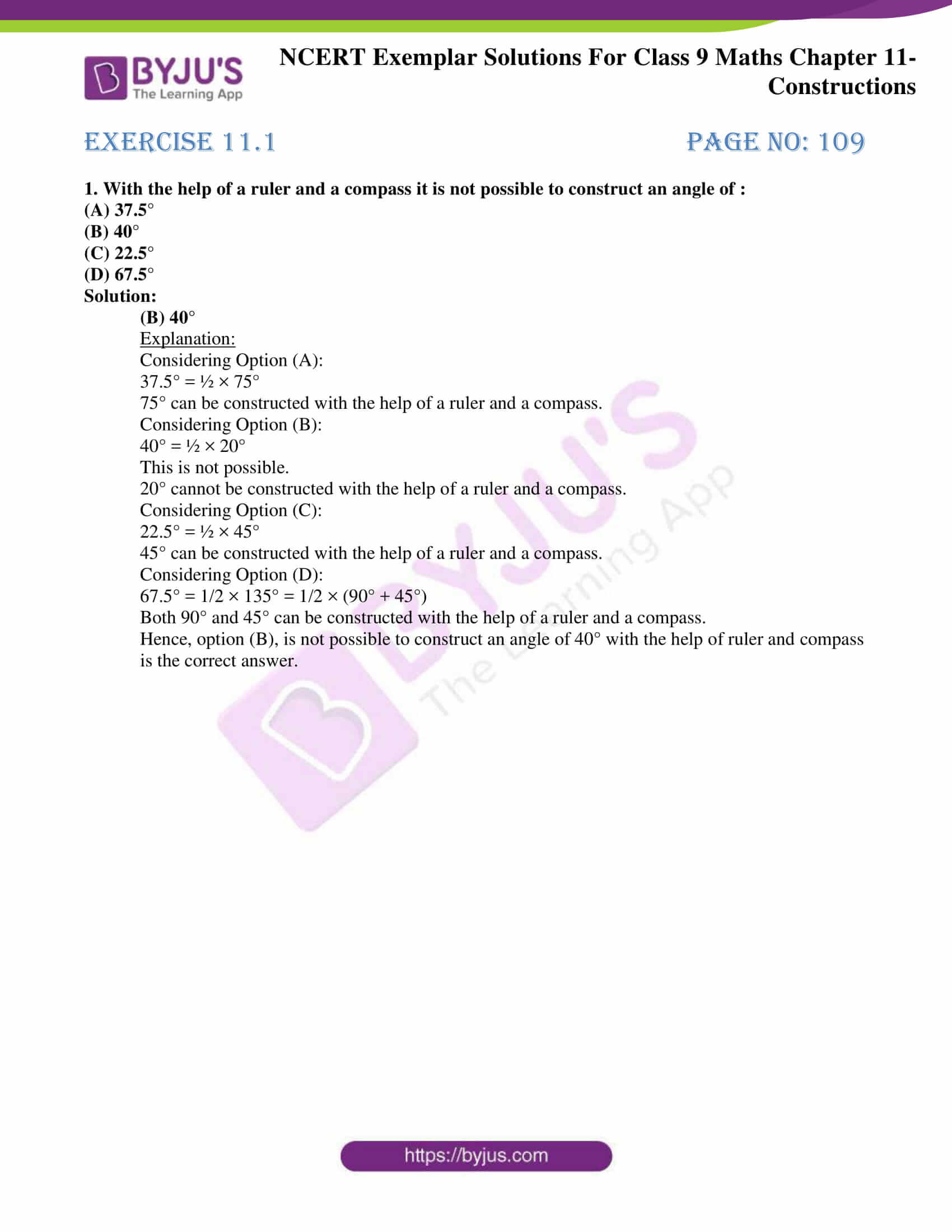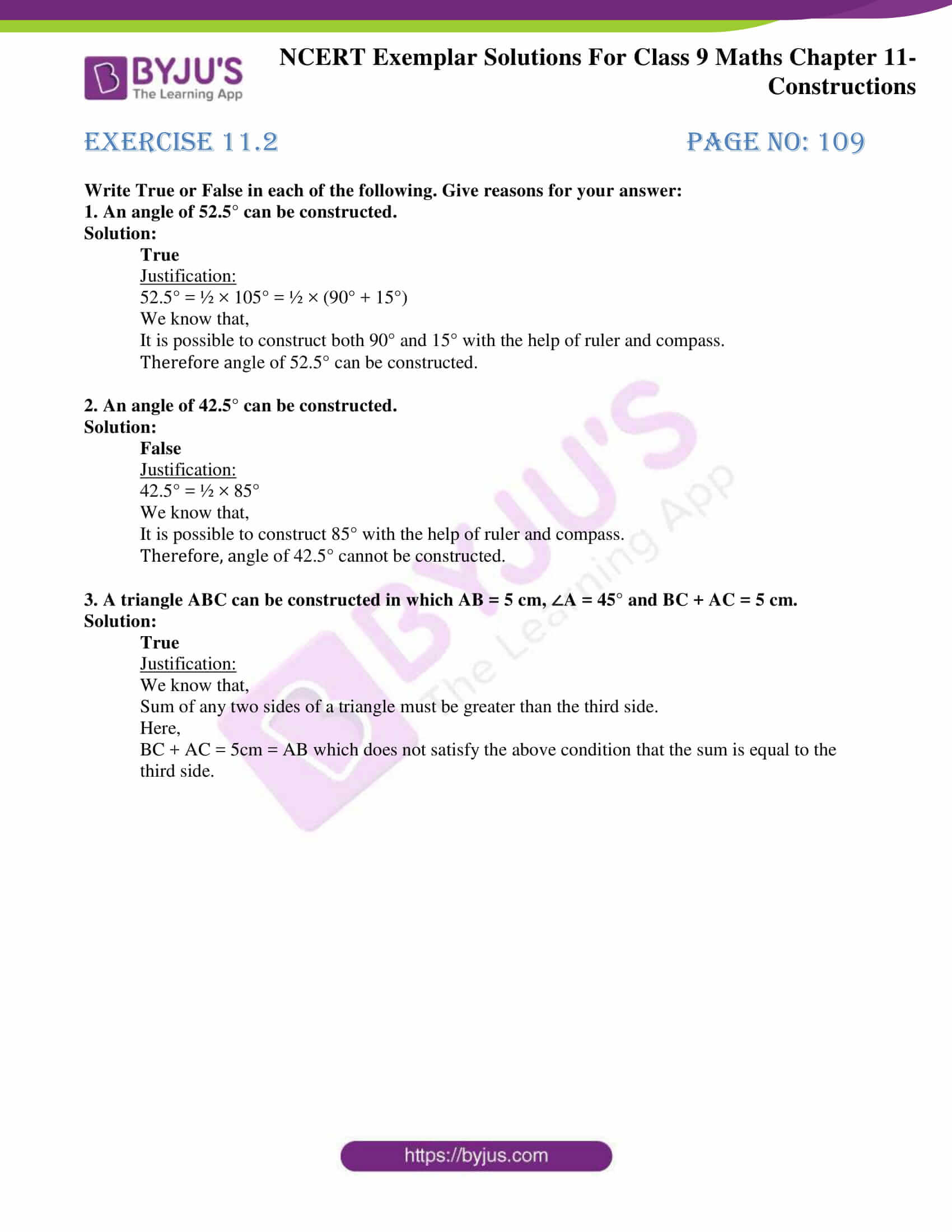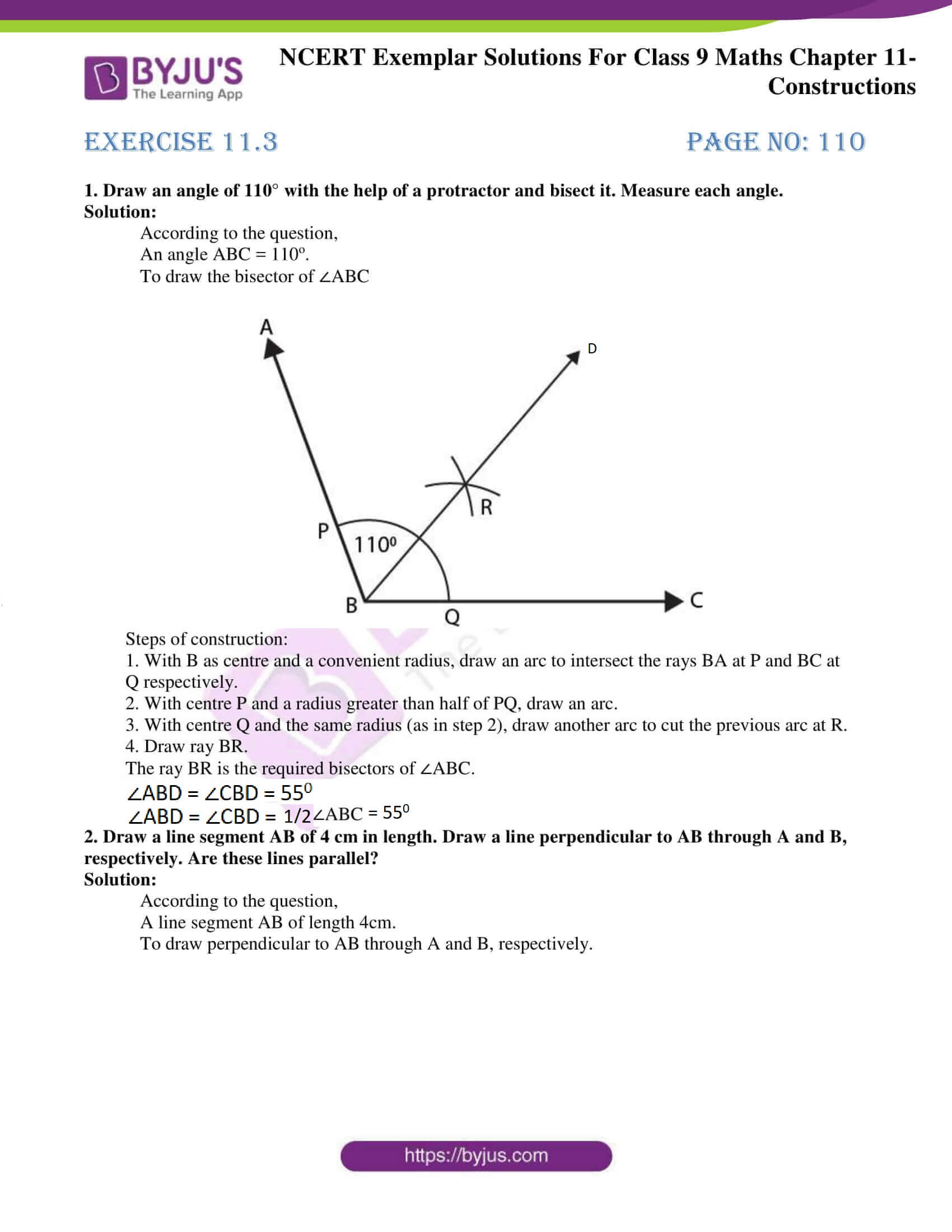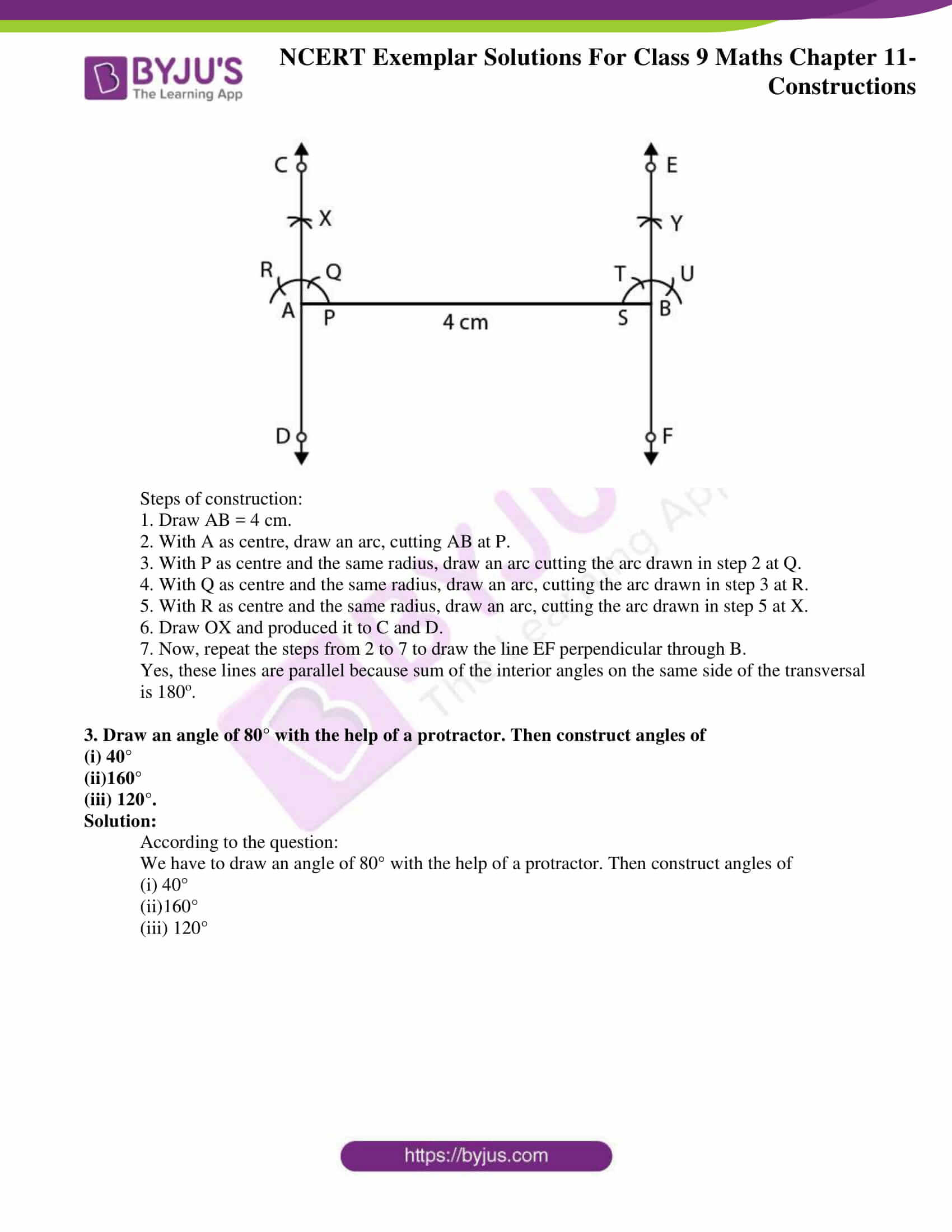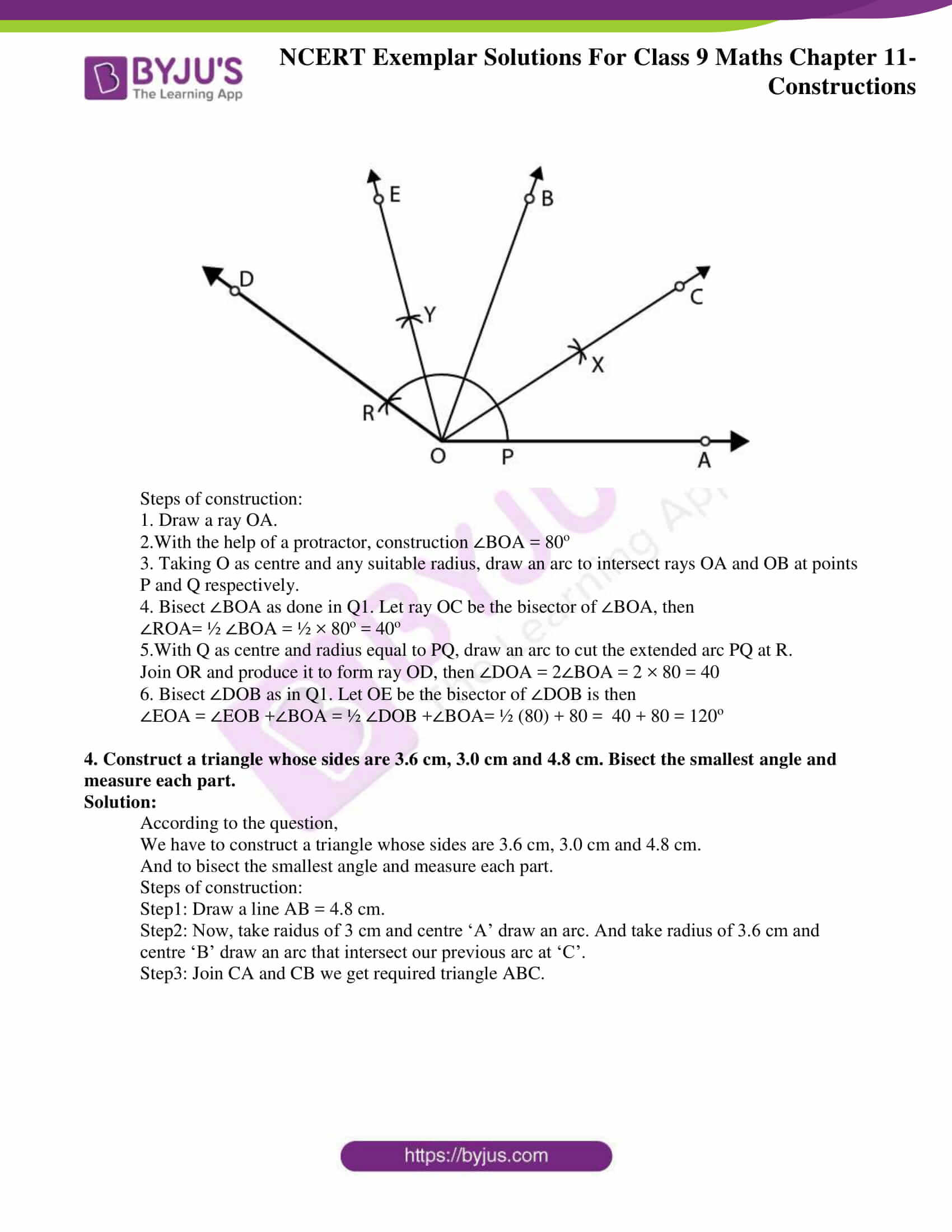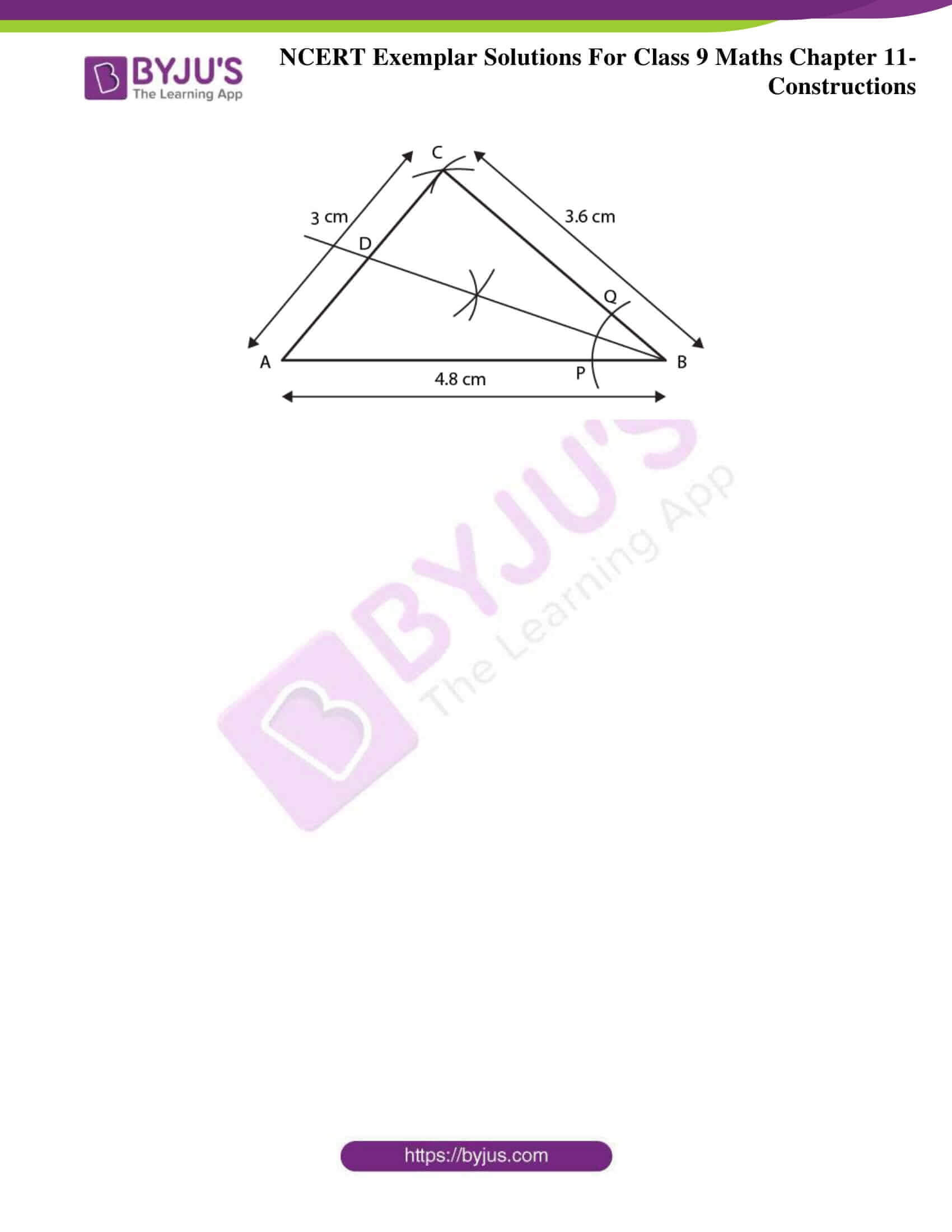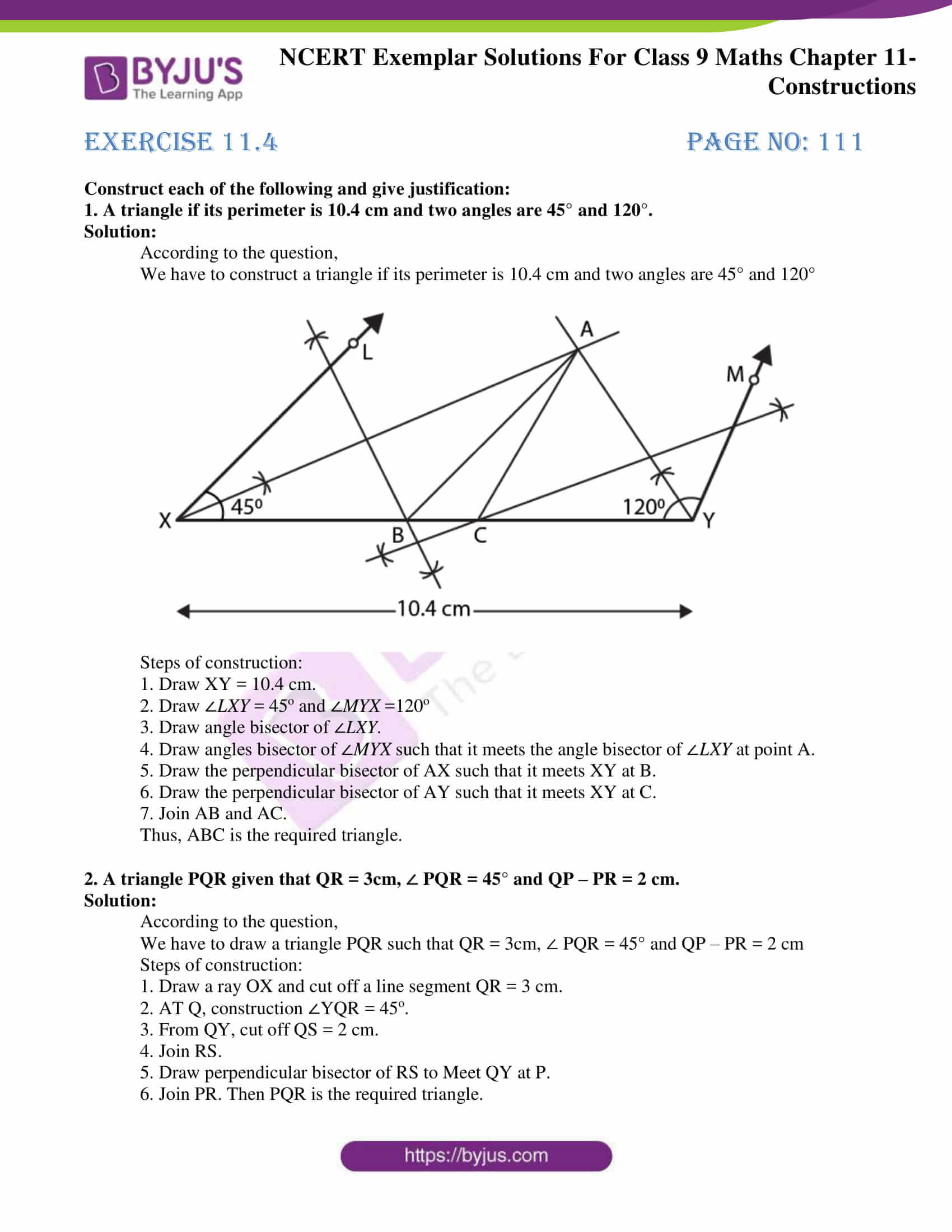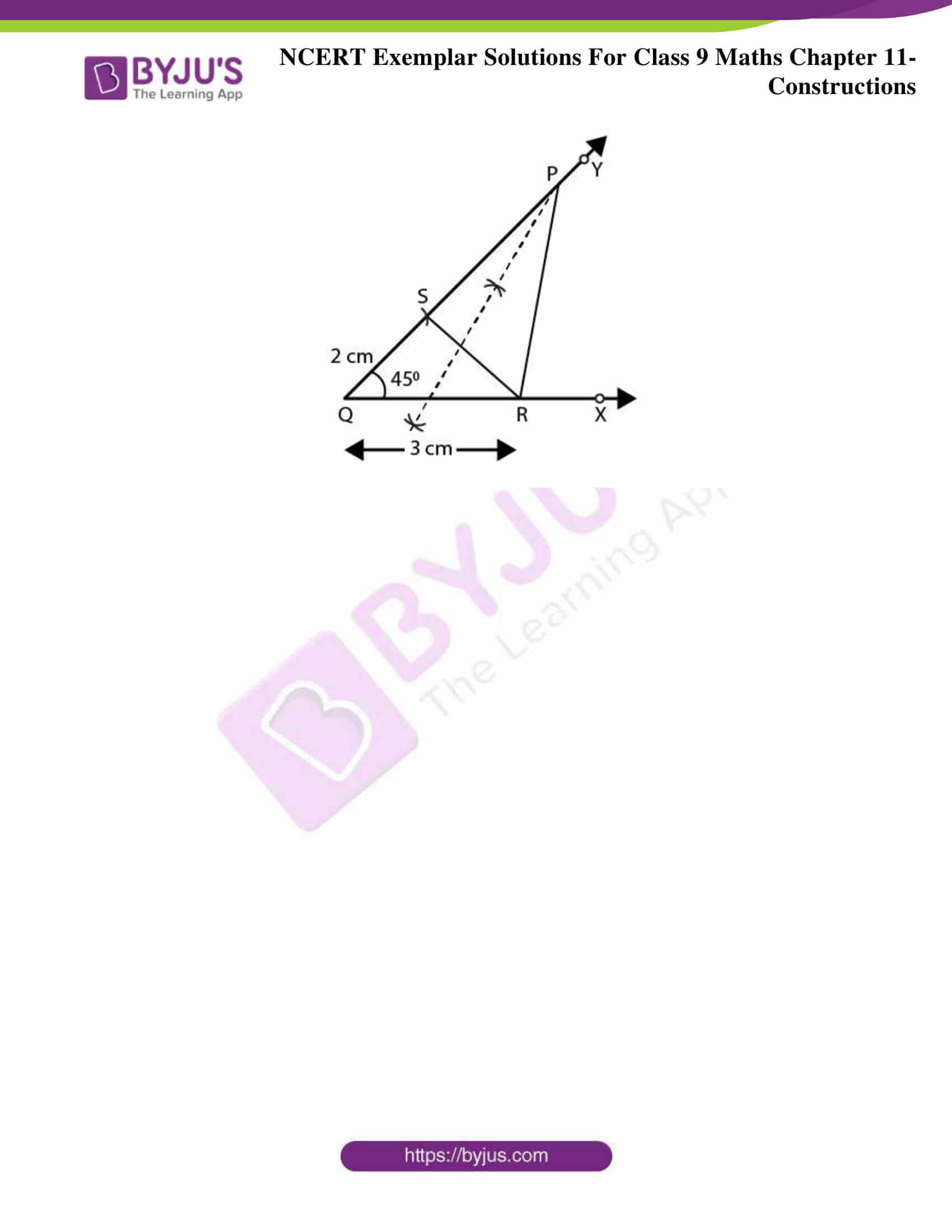### Access answers to NCERT Exemplar Solutions for Class 9 Maths Chapter 11 Circles

Exercise 11.1 Page No: 109

1. With the help of a ruler and a compass it is not possible to construct an angle of :

(A) 37.5°

(B) 40°

(C) 22.5°

(D) 67.5°

Solution:

(B) 40°

Explanation:

Considering Option (A):

37.5° = ½ × 75°

75° can be constructed with the help of a ruler and a compass.

Considering Option (B):

40° = ½ × 20°

This is not possible.

20° cannot be constructed with the help of a ruler and a compass.

Considering Option (C):

22.5° = ½ × 45°

45° can be constructed with the help of a ruler and a compass.

Considering Option (D):

67.5° = 1/2 × 135° = 1/2 × (90° + 45°)

Both 90° and 45° can be constructed with the help of a ruler and a compass.

Hence, option (B), is not possible to construct an angle of 40° with the help of ruler and compass is the correct answer.

Exercise 11.2 Page No: 109

Write True or False in each of the following. Give reasons for your answer:

1. An angle of 52.5° can be constructed.

Solution:

True

Justification:

52.5° = ½ × 105° = ½ × (90° + 15°)

We know that,

It is possible to construct both 90° and 15° with the help of ruler and compass.

Therefore angle of 52.5° can be constructed.

2. An angle of 42.5° can be constructed.

Solution:

False

Justification:

42.5° = ½ × 85°

We know that,

It is possible to construct 85° with the help of ruler and compass.

Therefore, angle of 42.5° cannot be constructed.

3. A triangle ABC can be constructed in which AB = 5 cm, ∠A = 45° and BC + AC = 5 cm.

Solution:

True

Justification:

We know that,

Sum of any two sides of a triangle must be greater than the third side.

Here,

BC + AC = 5cm = AB which does not satisfy the above condition that the sum is equal to the third side.

Exercise 11.3 Page No: 110

1. Draw an angle of 110° with the help of a protractor and bisect it. Measure each angle.

Solution:

According to the question,

An angle ABC = 110o.

To draw the bisector of ∠ABC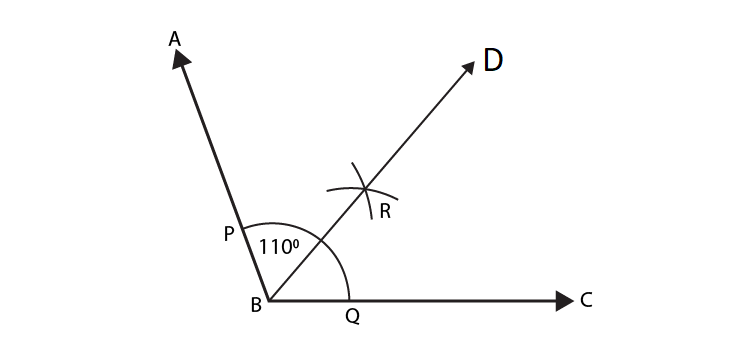Steps of construction:

1. With B as centre and a convenient radius, draw an arc to intersect the rays BA at P and BC at Q respectively.

2. With centre P and a radius greater than half of PQ, draw an arc.

3. With centre Q and the same radius (as in step 2), draw another arc to cut the previous arc at R.

4. Draw ray BR.

The ray BR is the required bisectors of ∠ABC.

∠ABD = ∠CBD = 550

∠ABD = ∠CBD = 1/2 ∠ABC = 1/2 X 1100 = 550

2. Draw a line segment AB of 4 cm in length. Draw a line perpendicular to AB through A and B, respectively. Are these lines parallel?

Solution:

According to the question,

A line segment AB of length 4cm.

To draw perpendicular to AB through A and B, respectively.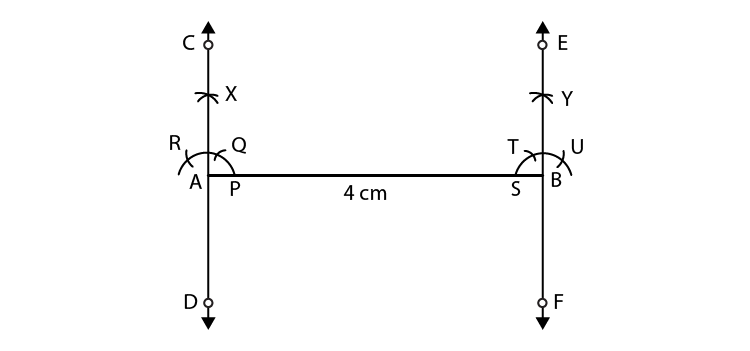Steps of construction:

1. Draw AB = 4 cm.

2. With A as centre, draw an arc, cutting AB at P.

3. With P as centre and the same radius, draw an arc cutting the arc drawn in step 2 at Q.

4. With Q as centre and the same radius, draw an arc, cutting the arc drawn in step 3 at R.

5. With R as centre and the same radius, draw an arc, cutting the arc drawn in step 5 at X.

6. Draw OX and produced it to C and D.

7. Now, repeat the steps from 2 to 7 to draw the line EF perpendicular through B.

Yes, these lines are parallel because sum of the interior angles on the same side of the transversal is 180o.

3. Draw an angle of 80° with the help of a protractor. Then construct angles of

(i) 40°

(ii)160°

(iii) 120°.

Solution:

According to the question:

We have to draw an angle of 80° with the help of a protractor. Then construct angles of

(i) 40°

(ii)160°

(iii) 120°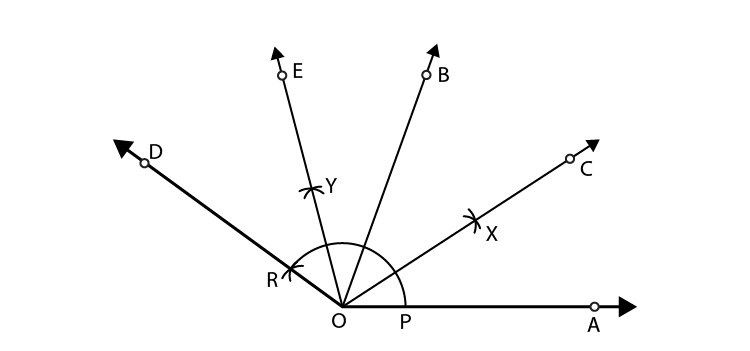Steps of construction:

1. Draw a ray OA.

2.With the help of a protractor, construction ∠BOA = 80o

3. Taking O as centre and any suitable radius, draw an arc to intersect rays OA and OB at points P and Q respectively.

4. Bisect ∠BOA as done in Q1. Let ray OC be the bisector of ∠BOA, then

∠ROA= ½ ∠BOA = ½ × 80o = 40o

5.With Q as centre and radius equal to PQ, draw an arc to cut the extended arc PQ at R.

Join OR and produce it to form ray OD, then ∠DOA = 2∠BOA = 2 × 80 = 40

6. Bisect ∠DOB as in Q1. Let OE be the bisector of ∠DOB is then

∠EOA = ∠EOB +∠BOA = ½ ∠DOB +∠BOA= ½ (80) + 80 = 40 + 80 = 120o

4. Construct a triangle whose sides are 3.6 cm, 3.0 cm and 4.8 cm. Bisect the smallest angle and measure each part.

Solution:

According to the question,

We have to construct a triangle whose sides are 3.6 cm, 3.0 cm and 4.8 cm.

And to bisect the smallest angle and measure each part.

Steps of construction:

Step1: Draw a line AB = 4.8 cm.

Step2: Now, take raidus of 3 cm and centre ‘A’ draw an arc. And take radius of 3.6 cm and

centre ‘B’ draw an arc that intersect our previous arc at ‘C’.

Step3: Join CA and CB we get required triangle ABC.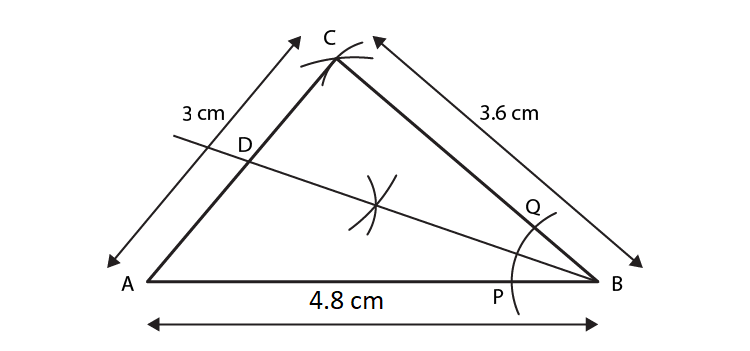Exercise 11.4 Page No: 111

Construct each of the following and give justification:

1. A triangle if its perimeter is 10.4 cm and two angles are 45° and 120°.

Solution:

According to the question,

We have to construct a triangle if its perimeter is 10.4 cm and two angles are 45° and 120°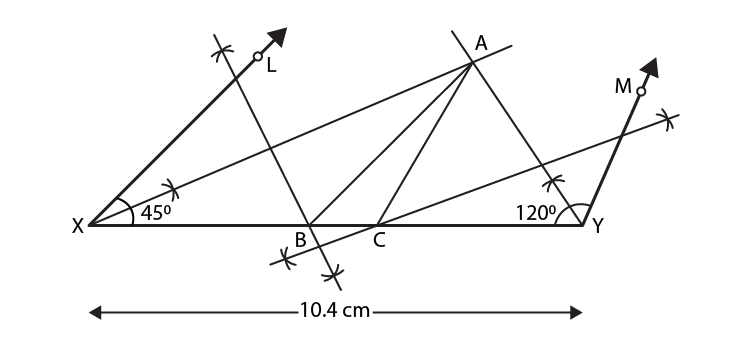Steps of construction:

1. Draw XY = 10.4 cm.

2. Draw ∠LXY = 45o and ∠MYX =120o

3. Draw angle bisector of ∠LXY.

4. Draw angles bisector of ∠MYX such that it meets the angle bisector of ∠LXY at point A.

5. Draw the perpendicular bisector of AX such that it meets XY at B.

6. Draw the perpendicular bisector of AY such that it meets XY at C.

7. Join AB and AC.

Thus, ABC is the required triangle.

2. A triangle PQR given that QR = 3cm, ∠ PQR = 45° and QP – PR = 2 cm.

Solution:

According to the question,

We have to draw a triangle PQR such that QR = 3cm, ∠ PQR = 45° and QP – PR = 2 cm

Steps of construction:

1. Draw a ray OX and cut off a line segment QR = 3 cm.

2. AT Q, construction ∠YQR = 45o.

3. From QY, cut off QS = 2 cm.

4. Join RS.

5. Draw perpendicular bisector of RS to Meet QY at P.

6. Join PR. Then PQR is the required triangle.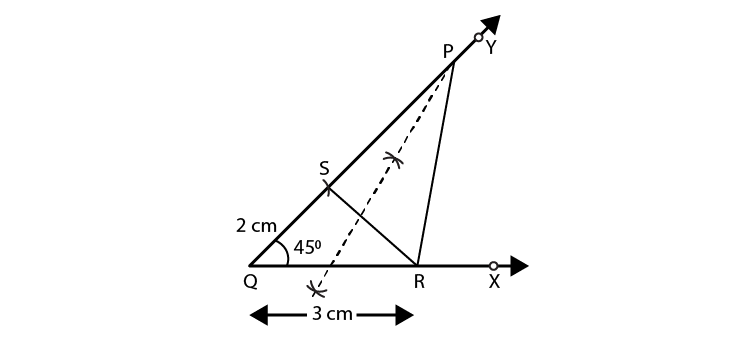Download BYJU’S app to get personalized video content and learn the concepts of maths such as constructions of different types of figures using geometry box, in an easy way.

## Frequently Asked Questions on NCERT Solutions for Class 11 Maths Chapter 11

### What are the topics covered under Chapter 11 of NCERT Exemplar Solutions for Class 9 Maths?

The following topics of constructions chapter are covered here;
1. Constructions of figures using geometry box instruments such as scale, set-squares, dividers, compass and protector.
2. Basic constructions such as bisector of a given angle, the perpendicular bisector of a given line segment and an angle at ray
3. Construction of triangle with given base, angles and the sides

### How to score good marks in the Chapter 11 of NCERT Exemplar Solutions for Class 9 Maths?

The Chapter 11 Constructions is important in Class 9 Mathematics. Once students cover the core areas, it will be easier for them to solve the exercise wise problems. Solving the problems related to this chapter will improve the analytical and logical thinking skills of students. Choosing the right study material also plays an important role in scoring good marks in the Class 9 exam. The main aim of creating the NCERT Exemplar Solutions is to help students analyse the concepts which they are lagging behind and work on them for a better score.

### Does the BYJU’S NCERT Exemplar Solutions for Class 9 Maths Chapter 11 provide accurate solutions as per the CBSE syllabus?

When it comes to Mathematics, accuracy is an important factor and regular practice is required to achieve that. As there are various study materials available online, selecting the correct one is a challenging task. By opting for the solutions which completely focus on the CBSE syllabus, students will be able to understand the concepts which are important from the exam perspective.